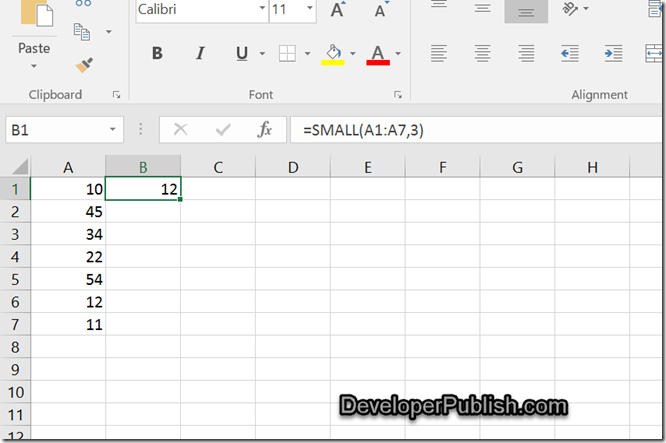# How to find the Nth Smallest Number from a List of values in Excel ?

In one of the previous blog posts , we saw how to get the Nth largest number from a list of values in Excel. In this blog post , lets look at getting the Nth smallest value from the list.

# How to find the Nth Smallest Number from a List of values in Excel ?

Microsoft Excel provides the function SMALL which lets you to this.

The function LARGE takes 2 parameters.
– The first parameter takes the range of cells.
– The second parameter specifies the nth smallest value that you are searching for.

Assume that the Excel Column (A1:A7) contains the range of values , you would need to find the 3rd Largest value from this range and display it in the cell B1.

Just enter the following formula in the cell B1.

=SMALL(A1:A7,3)